NEET  >  NEET Previous Year Questions (2014-22): Wave Optics

# NEET Previous Year Questions (2014-22): Wave Optics - Notes | Study Physics Class 12 - NEET

 1 Crore+ students have signed up on EduRev. Have you?

Q.1. In a Young’s double slit experiment, a student observes 8 fringes in a certain segment of screen when a monochromatic light of 600 nm wavelength is used. If the wavelength of light is changed to 400 nm, then the number of fringes he would observe in the same region of the screen is                   (2022)
(1) 8
(2) 9
(3) 12
(4) 6
Ans: (3)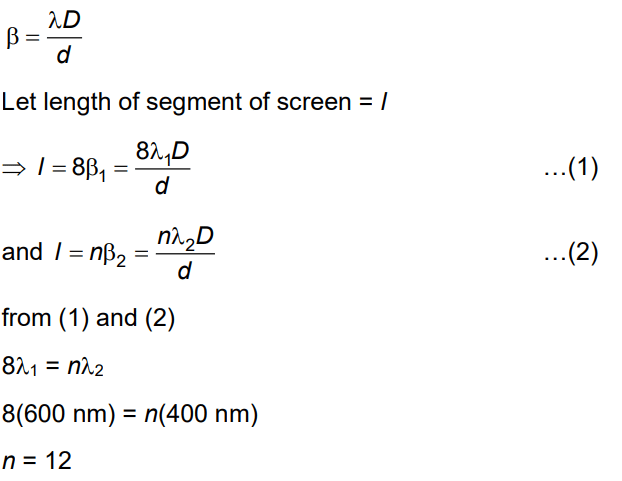Q.2. Assume that light of wavelength 600 nm is coming from a star. The limit of resolution of telescope whose objective has a diameter of 2m is:      (2020)

Ans:
C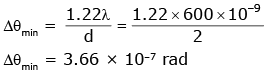Q.3. The Brewster’s angle ib for an interface should be :       (2020)
A: 45° < ib < 90°
B: ib = 90°
C: 0° < ib < 30°
D: 30° < ib < 45°
Ans:
A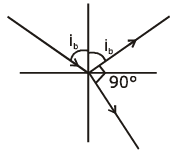m = tan (ib)
ib =  tan-1 (μ)
(μ > 1)
ib > 45
45 < ib < 90°

Q.4. In Young's double slit experiment, if the separation between coherent sources is halved and the distance of the screen from the coherent sources is doubled, then the fringe width becomes :       (2020)
A: four times

B: one-fourth
C: double
D: half

Ans: A
Fringewidth in YDSE is given by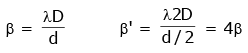Q.5. In a double slit experiment, when light of wavelength 400 nm was used, the angular width of the first minima formed on a screen placed 1 m away, was found to be 0.2°. What will be the angular width of the first minima, if the entire experimental apparatus is immersed in water? (μwater = 4/3)    (2019)
A: 0.266°
B: 0.15°
C: 0.05°
D: 0.1°
Ans:
B
Solution:
In air angular fringe width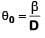Angular fringe width in water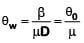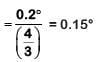Q.6. Unpolarised light is incident from air on a plane surface of a material of refractive index 'μ'. At a particular angle of incidence 'i', it is found that the reflected and refracted rays are perpendicular to each other. Which of the following options is correct for this situation ?    (2018)
A: Reflected light is polarised with its electric vector parallel to the plane of incidence

B: Reflected light is polarised with its electric vector perpendicular to the plane of incidence
C: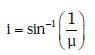D: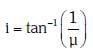Ans: B
Solution: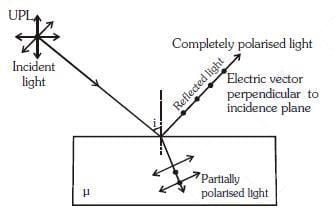Q.7. In Young's double slit experiment the separation between the slits is 2 mm, the wavelength λ of the light used is 5896 Å and distance D between the screen and slits is 100 cm. It is found that the angular width of the fringes is 0.20°. To increase the fringe angular width to 0.21° (with same λ and D) the separation between the slits needs to be changed to :-    (2018)
A: 1.8 mm
B: 1.9 mm
C: 2.1 mm
D: 1.7 mm

Ans: B
Solution: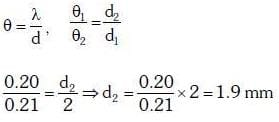Q.8. Young's double slit experiment is first performed in air and then in a medium other than air. It is found that 8th bright fringe in the medium lies where 5th dark fringe lies in air. The refractive index of the medium is nearly :-    (2017)
A: 1.59
B: 1.69
C: 1.78
D: 1.25
Ans:
C
Solution:

Fringe Width in a medium -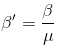- where in
Since wavelength in a medium become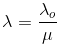Fringe width in air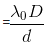Fringe width in any other medium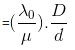According to question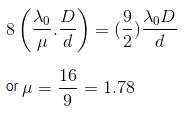Q.9. Two Polaroids P1 and P2 are placed with their axis perpendicular to each other. Unpolarised light I0 is incident on P1 . A third polaroid P3 is kept in between P1 and P2 such that its axis makes an angle 45° with that of P1. The intensity of transmitted light through P2 is :-    (2017)
A: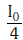B: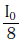C: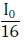D: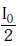Ans:
B
Solution: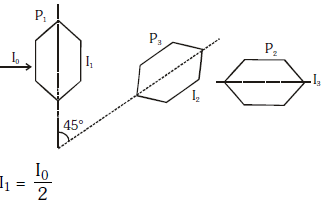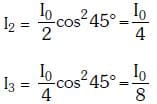Q.10. In a diffraction pattern due to a single slit of width 'a' the first minimum is observed at an angle 30º when light of wavelength 5000 Å is incident on the slit. The first secondary maximum is observed at an angle of :    (2016)
A: sin-1 (3/4)
B: sin-1 (1/4)
C: sin-1 (2/3)
D: sin-1 (3/2)
Ans:
A
Solution:

Given that, first minimum is observed at an angle of 30º in a diffraction pattern due to a single slit of width a.
i.e., n = 1, θ = 30º
According to the Bragg's law of diffraction,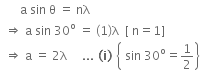For 1st secondary maxima,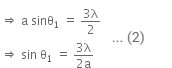Putting the value of a from Eqn. (i) to Eqn. (ii), we get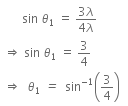Q.11. The intensity at the maximum in Young's double slit experiment is I0. Distance between two slits is d = 5λ, where λ is the wavelength of light used is the experiment. What will be the intensity in front of one of the slits on the screen placed at a distance D = 10d ?    (2016)
A: I0/2
B: I0
C: I0/4
D: 3I0/4
Ans:
A
Solution:

Given,
Maximum intensity = Io
Distance between the slits, d = 5λ
Distance of screen from the slit, D = 10d
Using the formula,
Path difference,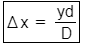Here,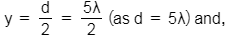D = 10d = 50λ
So,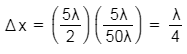Corresponding phase difference will be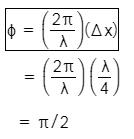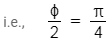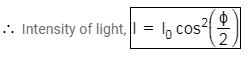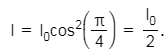Q.12. A radiation of energy = ‘E’ falls normally on a perfectly reflecting surface. The momentum transferred to the surface is (C = Velocity of light) :    (2015)
A: E/C2
B: E/C
C: 2E/C
D: 2E/C2
Ans:
C
Solution:

The radiation energy is given by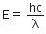Initial momentum of the radiation is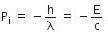The reflected momentum is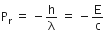So, the change in momentum of light is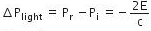Thus, the momentum transferred to the surface is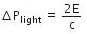Q.13. In a double slit experiment, the 2 slits are 1 mm apart and the screen is placed 1 m away. A monochromatic light of wavelength 500 nm is used. What will be the width of each slit for obtaining ten maxima of double slit within the central maxima of single slit pattern ?    (2015)
A: 0.02 mm
B: 0.2 mm
C: 0.1 mm
D: 0.5 mm
Ans:
B
Solution:

In a double slit experiment, the two slits are 1 mm apart.
d = 1 mm = 10-3 m.
The screen is placed at a distance D = 1 m away. Monochromatic light of wave length
λ = 500 nm = 5 x 10-7 m is used.
The distance between two successive maxima or two successive minima is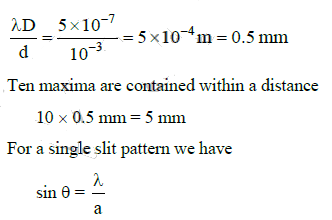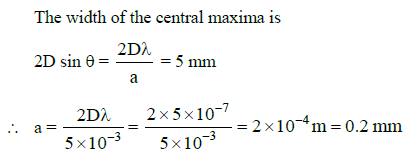Q.14. For a parallel beam of monochromatic light of wavelength ‘λ’, diffraction is produced by a single slit whose width ‘a’ is of the order of the wavelength of the light. If ‘D’ is the distance of the screen from the slit, the width of the central maxima will be :    (2015)
A: 2Da/λ
B: 2Dλ/a
C: Dλ/a
D: Da/λ
Ans: B
Solution:

For a parallel beam of monochromatic light of wavelength λ, diffraction is produced by a single slit whose width 'a' is of the order of the wavelength we have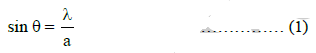where θ is the angle subtended by the first minima and the central maxima at the slit.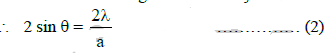If x is the width of the central maxima, we have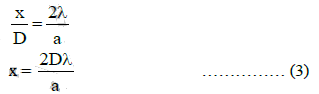where D is the distance of the screen from the slit

Q.15. A beam of light of λ = 600 nm from a distant source falls on a single slit 1mm wide and the resulting diffraction pattern is observed on a screen 2m away. The distance between first dark fringes on either side of the central bright fringe is :    (2014)
A: 2.4 cm
B: 2.4 mm
C: 1.2 cm
D: 1.2 mm
Ans:
B
Solution: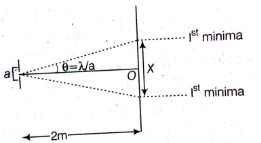From the above figure,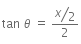For small θ and when θ is counted in rad, tan ≈ θ

So,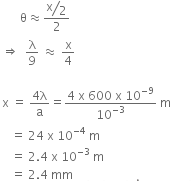Q.16. In the Young’s double−slit experiment, the intensity of light at a point on the screen where the path difference is λ is K, (λ being the wave length of light used). The intensity at a point where the path difference is λ/4, will be :    (2014)
A: K/2
B: Zero
C: K
D: K/4
Ans: A
Solution:

I = 4Io cos2(S/2)
4I0 = K
∵ δ = 2π if path different = λ
Phase difference when path difference = Δ/4 is equal to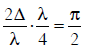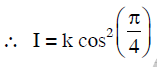"There is no Question for NEET 2021"

The document NEET Previous Year Questions (2014-22): Wave Optics - Notes | Study Physics Class 12 - NEET is a part of the NEET Course Physics Class 12.
All you need of NEET at this link: NEET

## Physics Class 12

157 videos|452 docs|213 tests
 Use Code STAYHOME200 and get INR 200 additional OFF

## Physics Class 12

157 videos|452 docs|213 tests

### How to Prepare for NEET

Read our guide to prepare for NEET which is created by Toppers & the best Teachers

Track your progress, build streaks, highlight & save important lessons and more!

,

,

,

,

,

,

,

,

,

,

,

,

,

,

,

,

,

,

,

,

,

;